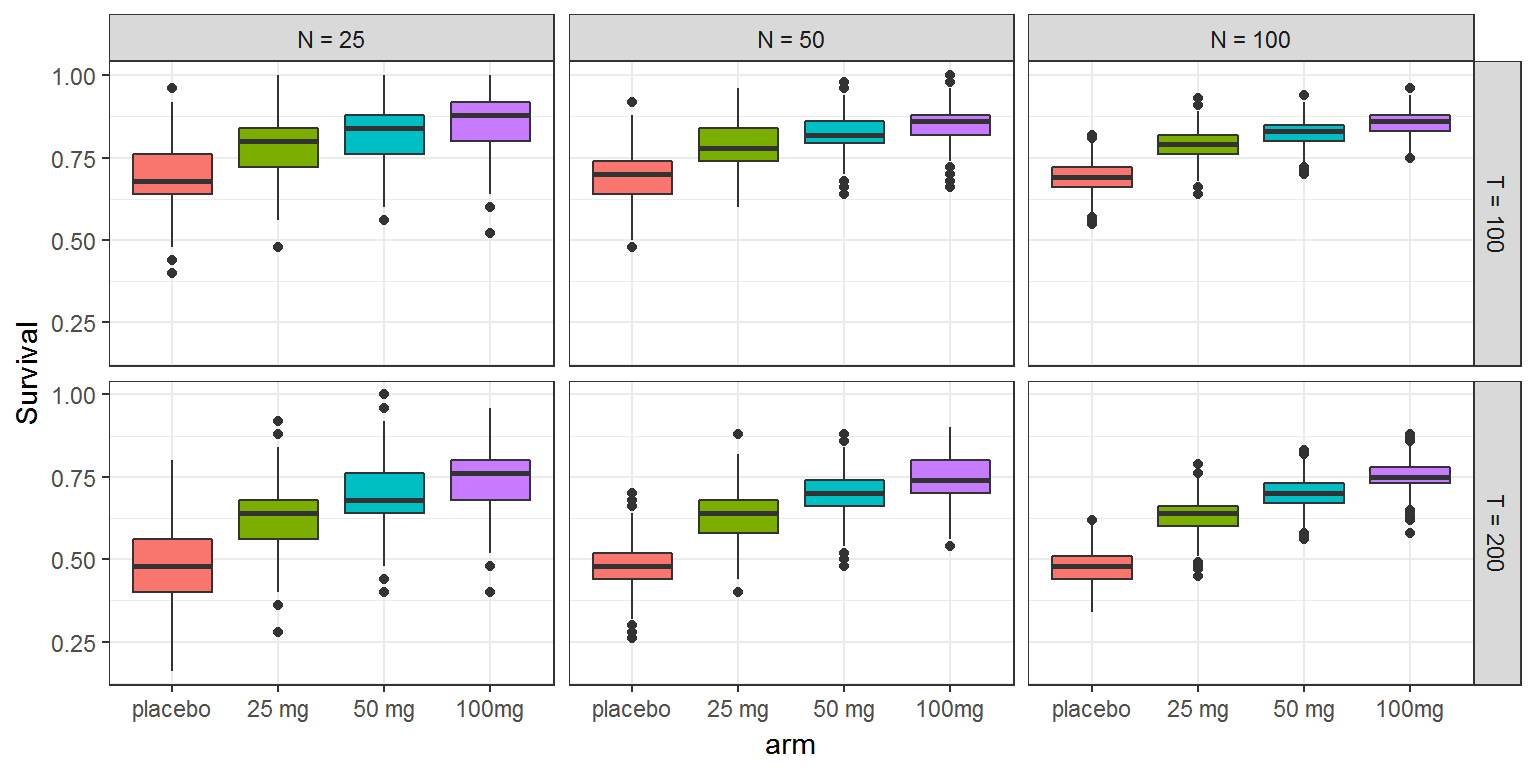$$\newcommand{\esp}{\mathbb{E}\left(#1\right)} \newcommand{\var}{\mbox{Var}\left(#1\right)} \newcommand{\deriv}{\dot{#1}(t)} \newcommand{\prob}{ \mathbb{P}\!(#1)} \newcommand{\eqdef}{\mathop{=}\limits^{\mathrm{def}}} \newcommand{\by}{\boldsymbol{y}} \newcommand{\bc}{\boldsymbol{c}} \newcommand{\bpsi}{\boldsymbol{\psi}} \def\pmacro{\texttt{p}} \def\like{{\cal L}} \def\llike{{\cal LL}} \def\logit{{\rm logit}} \def\probit{{\rm probit}} \def\one{{\rm 1\!I}} \def\iid{\mathop{\sim}_{\rm i.i.d.}} \def\simh0{\mathop{\sim}_{H_0}} \def\df{\texttt{df}} \def\res{e} \def\xomega{x} \newcommand{\argmin}{{\rm arg}\min_{#1}} \newcommand{\argmax}{{\rm arg}\max_{#1}} \newcommand{\Rset}{\mbox{\mathbb{R}}} \def\param{\theta} \def\setparam{\Theta} \def\xnew{x_{\rm new}} \def\fnew{f_{\rm new}} \def\ynew{y_{\rm new}} \def\nnew{n_{\rm new}} \def\enew{e_{\rm new}} \def\Xnew{X_{\rm new}} \def\hfnew{\widehat{\fnew}} \def\degree{m} \def\nbeta{d} \newcommand{\limite}{\mathop{\longrightarrow}\limits_{#1}} \def\ka{k{a}} \def\ska{k{\scriptscriptstyle a}} \def\kel{k{e}} \def\skel{k{\scriptscriptstyle e}} \def\cl{C{\small l}} \def\Tlag{T\hspace{-0.1em}{lag}} \def\sTlag{T\hspace{-0.07em}{\scriptscriptstyle lag}} \def\Tk{T\hspace{-0.1em}{k0}} \def\sTk{T\hspace{-0.07em}{\scriptscriptstyle k0}} \def\thalf{t{1/2}} \newcommand{\Dphi}{\partial_\pphi #1} \def\asigma{a} \def\pphi{\psi} \newcommand{\stheta}{{\theta^\star}} \newcommand{\htheta}{{\widehat{\theta}}}$$

The aim of this third part is to assess the information we can derive from a clinical trial with a particular design. One of the main characteristics of a clinical trial is that we have between-patient variation and a finite number of patients. Indeed, even if a treatement as an effect on survival as we have demonstrated in part I and part II of this example, a clinical trial with a limited number of patients will not necessarily be able to demonstrate this effect if a bad design has been selected.

It is therefore critical to assess the power of the trial. Here we show how we can compute the power by simulation of clinical trials with different number of patients and how we can compare the empirical survivals and the probability to conclude, correctly, that the treatment is effective.

# The PKPD model

We use the same PKPD model as in part II. To have a more realistic scenario an observational error is added to the predicted concentration. This is done with an additional block DEFINITION in section [LONGITUDINAL] where the probability distributions of the outcomes are described.

We use here a normal distribution for the continuous outcome (concentration) and a survival model for the event. Survival is defined by a single event so the parameter maxEventNumber has to be set to one. The study has a finite duration. This means that we can not make any conclusions about survival after the study finished at 200 hours. In effect, this is a right censoring of the data and the parameter rightCensoringTime is set accorindly to 200.

This new joint PKPD model is implemented in the text file model/cts2III.txt:

[LONGITUDINAL]
input={Tk0,V,Cl,Imax,E0,IC50,kout,alpha,beta,b}

EQUATION:
C = pkmodel(Tk0, V, Cl)
g = b*C

E_0 = E0
kin = E0*kout
ddt_E = kin*(1-Imax*C/(C+IC50)) - kout*E

h = (alpha/1000)*exp(beta*E)

DEFINITION:
y = {distribution=normal, prediction=C, sd=g}
e = {type=event, maxEventNumber=1, rightCensoringTime=200, hazard=h}

;-----------------------------------------
[INDIVIDUAL]
input={Tk0_pop,V_pop,Cl_pop,Imax_pop,E0_pop,IC50_pop,kout_pop,alpha_pop,beta_pop,omega_Tk0,omega_V,omega_Cl,omega_Imax,omega_E0,omega_IC50,omega_kout,omega_alpha,omega_beta}

DEFINITION:
Tk0   = {distribution=lognormal,   prediction=Tk0_pop,  sd=omega_Tk0}
V     = {distribution=lognormal,   prediction=V_pop,    sd=omega_V}
Cl    = {distribution=lognormal,   prediction=Cl_pop,   sd=omega_Cl}
E0    = {distribution=lognormal,   prediction=E0_pop,   sd=omega_E0}
IC50  = {distribution=lognormal,   prediction=IC50_pop, sd=omega_IC50}
kout  = {distribution=lognormal,   prediction=kout_pop, sd=omega_kout}
Imax  = {distribution=logitnormal, prediction=Imax_pop, sd=omega_Imax}
alpha = {distribution=normal,      prediction=alpha_pop,sd=omega_alpha}
beta  = {distribution=normal,      prediction=beta_pop, sd=omega_beta}


# Clinical trial simulation

We investigate different trial designs different number of patients and different doses:

• $$N=25$$, 50, and 100 patients,
• repeated oral administrations every 12 hours with different amounts: 0mg, 25mg, 50mg, 100mg.

Each trial simulation will be different because of the between-patient variability and the observational error. To understand the variability between different trial simulations we simulate $$M=1000$$ replicates of each trial design, i.e. a total of $$3\times4\times1000=12\,000$$ trials.

M  <- 1000
vN <- c(25, 50, 100)
adm.amount <- c(0, 25, 50, 100)
adm.time   <- seq(0, 200, by=12)

Here, survival at t=100h and t=200h is the endpoint of interest. We will therefore use the event $$e$$ defined in the model with the summary statistics surv.t defined at times 100 and 200.

e <- list(name='e', time=0, surv.time=c(100,200))

We use the same PKPD population parameters for each simulation:

pop.param   <- c(
Tk0_pop   = 3,    omega_Tk0   = 0.2,
V_pop     = 10,   omega_V     = 0.2,
Cl_pop    = 1,    omega_Cl    = 0.2,
Imax_pop  = 0.8,  omega_Imax  = 0.5,
E0_pop    = 100,  omega_E0    = 0.1,
IC50_pop  = 4,    omega_IC50  = 0.1,
kout_pop  = 0.1,  omega_kout  = 0.1,
alpha_pop = 0.5,  omega_alpha = 0.1,
beta_pop  = 0.02, omega_beta  = 0,
b         = 0.1
)

We use simulx for simulating $$M$$ replicates of the trial, for each number of patients $$N$$ and each dose.

The survival rates at times 100h and 200h for each simulated trial are stored in an array R.

Important: In order to avoid any memory issue, we set settings$out.trt = F on purpose not to store the treatment given to each patient in each replicate. labels.arm <- c("placebo", "25 mg", "50 mg", "100mg") labels.N <- c("N = 25", "N = 50", "N = 100") R <- NULL ptm <- proc.time() for (k in seq(1,length(adm.amount))){ adm <- list(time=adm.time, amount=adm.amount[k]) for (l in seq(1,length(vN))){ g <- list(size=vN[l], level='individual'); res <- simulx(model = "model/cts2III.txt", parameter = pop.param, treatment = adm, output = e, group = g, nrep = M, settings = list(out.trt = F)) res$e$nbEv.mean <- NULL R <- rbind(R, cbind(res$e, N=labels.N[l], arm=labels.arm[k]))
}
}
computational.time <- proc.time() - ptm

Once all the results are stored in array R, we can compare the survivals at time $$t=100$$ and $$t=200$$ using boxplots:

library(reshape2)
rm <- melt(R,  id = c('N','arm','rep'), value.name="Survival", variable.name="time")
rm$time <- factor(rm$time, labels = c("T = 100", "T = 200"))

print(ggplot(rm, aes(arm,Survival)) + geom_boxplot(aes(fill = arm)) +
facet_grid(time ~N) + theme(legend.position="none"))Imagine now that we conclude that the treatment is efficient if the difference in observed survival between the control group and the test group is greater than 0.1 at time 200.

The results of the simulation can now be used for estimating the power of the study, i.e. the probability to conclude, correctly, that the treatment is efficient, according to the dose and the size of the trial (we expect that this probability increases with both the dose and the size if the treatment is indeed efficient in theory’’ ).

S <- 0.1

R.p <- R[R$arm=="placebo","S200.mean"] R.t <- R[R$arm!="placebo",]
R.t$dS <- (R.t$S200.mean-rep(R.p,3) > S)
R.t\$S200.mean <- NULL
print(acast(R.t,arm~N,mean, value.var="dS"))
##       N = 25 N = 50 N = 100
## 25 mg  0.657  0.669   0.790
## 50 mg  0.796  0.862   0.954
## 100mg  0.908  0.955   0.996

Let us display the total computational time for simulating 12 000 trials on a labtop:

print(computational.time)
##    user  system elapsed
##  760.97   30.09  213.97

part I     part II     part III